# Rubik's Cube

Can a wooden block, wrapped in LED strips be turned into Rubik's cube?

IntermediateShowcase (no instructions)2,035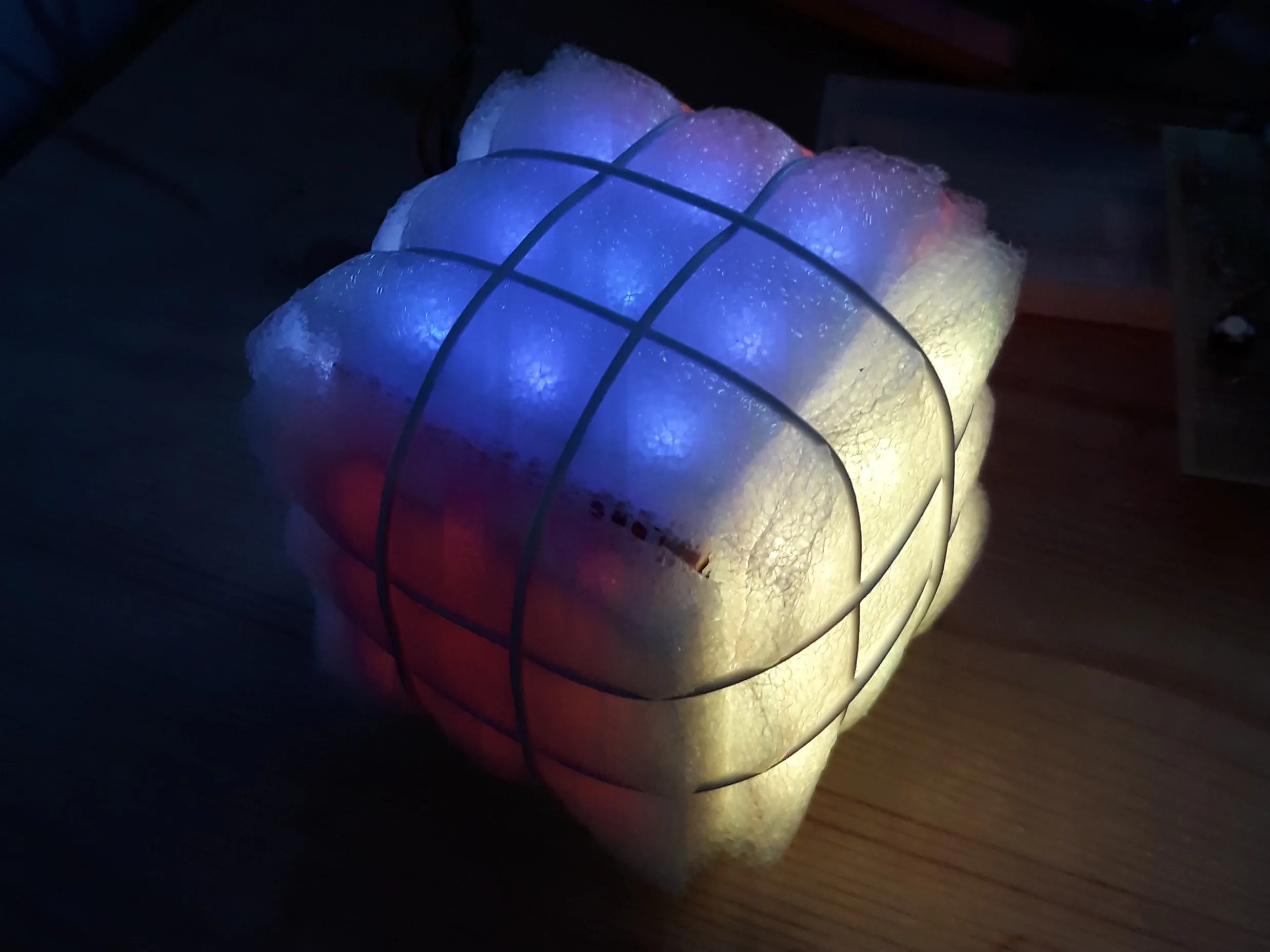## Things used in this project

### Hardware componentsArduino UNO & Genuino UNO
×1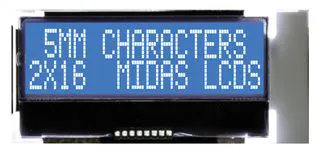Alphanumeric LCD, 16 x 2
×1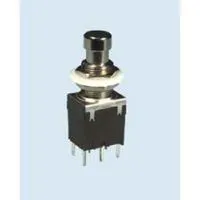Pushbutton Switch, Pushbutton
×8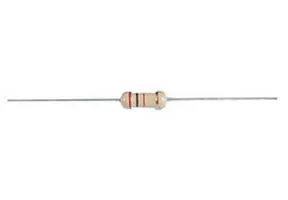Through Hole Resistor, 470 ohm
×1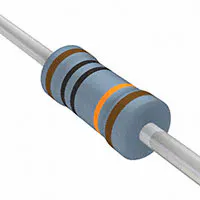Resistor 100k ohm
×2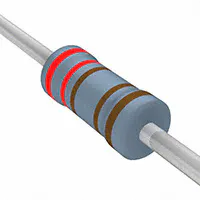Resistor 2.21k ohm
×10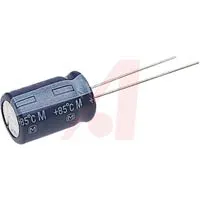Capacitor 10 µF
×1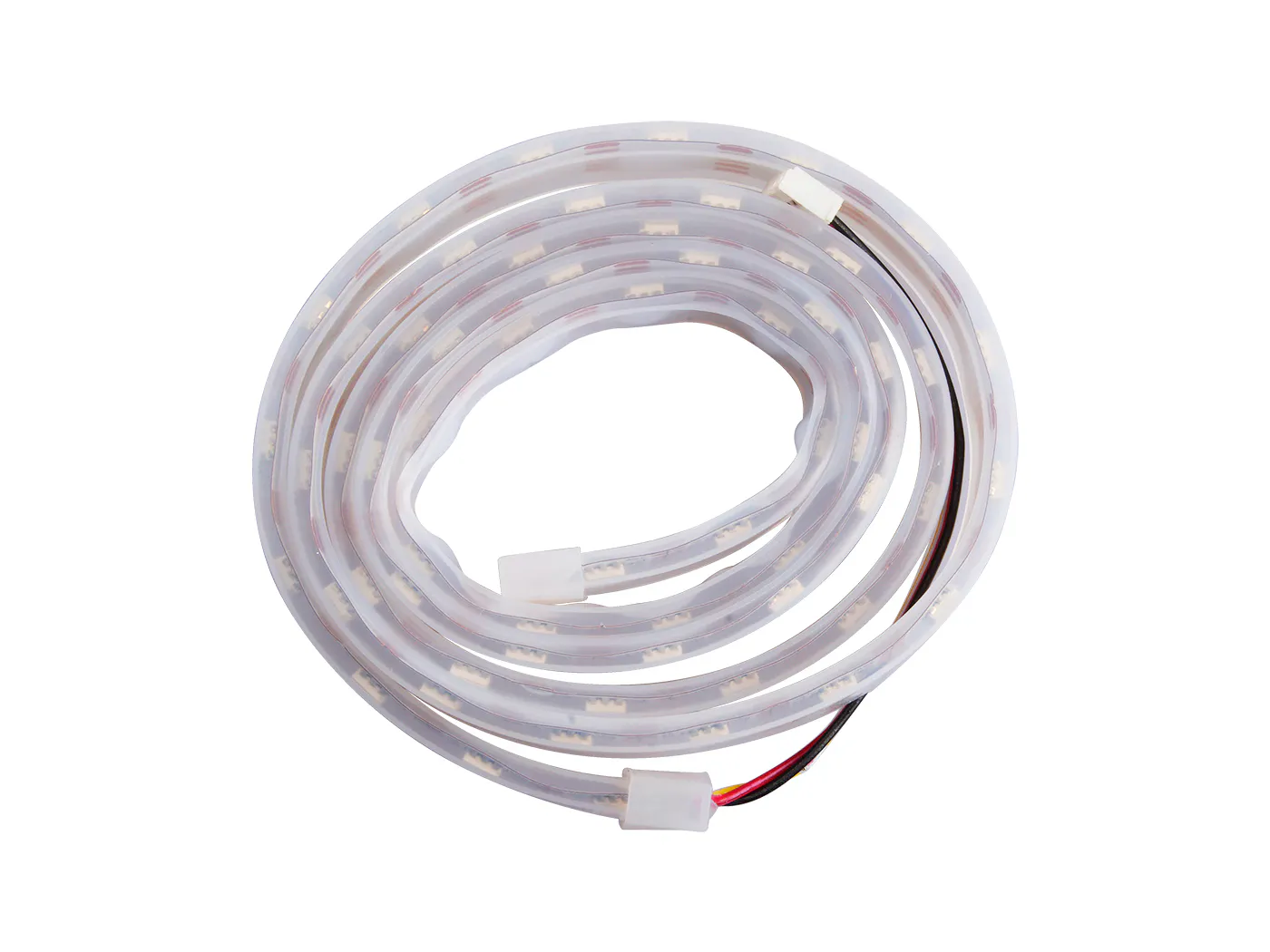Seeed Grove - WS2813 RGB LED Strip Waterproof - 60 LED/m - 1m
×1

### Hand tools and fabrication machinesSoldering iron (generic)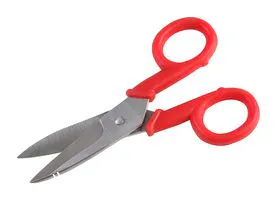Scissor, Electrician

## Code

### Rubik's Cube

C/C++
Main program
```/* this program turns a ledstrip glued to a wooden cube into a Rubiks Cube
*
*/
//major changes on March 18th 2019
#include "choices.h"
#include "cube.h"
#include "output.h"
#include <LiquidCrystal.h>
#include <PololuLedStrip.h>

Choices LeftPanel(A5, 5); //set 5 options at pin A5
Choices RightPanel(A4, 3);//set 3 options at pin A3
Cube MyCube(3);           //would be nice to have DO port of ledstrip as an argument
Output MyOutput(false, true);  //output to Serial output and or lcd output

#define _dutch   //dutch language selected, conditional compiling is applied because insufficient dynamic memory to store 2 languages

void setup() {
Serial.begin(9600);
delay(500);
MyCube.show();
delay(500);
MyOutput.clrscr();
#ifndef _dutch
MyOutput.txt("Hello there!");  //default language
#else
MyOutput.txt("Hallo daar!");
#endif
MyCube.littleShow();
MyOutput.clrscr();
}

const int turnSide  = 0;
const int turnMid   = 1;
const int turnCube  = 2;
const int undoLast  = 3;
const int solveCube = 4;

const bool CW = true;

const int histMax = 30;    //max number of turns in memory
int turnNr      = 0;       //number of turns done
int lastSolved  = 0;       //last time that cube was solved
int turnsInHist = 0;       //current number of turns in history
byte turnHist[histMax];
/* turns are stored in turnHist
*  1 byte is used to store turn
*  1 byte may represent numbers from 0 - 255
*  coding: 100*dir + 10 * side + task
*  history should be implemented in Class Cube
*/
bool escFlag = false;

void loop() {

int side;
bool dir;

escFlag = false;
case turnSide:
while (true) {
side = getSide();
if (escFlag) break;
dir  = getDir();
if (escFlag) break;
MyCube.showTurnSide(side, dir);
turnNr++;
turnsInHist++;
}
break;
case turnMid:
while (true) {
side = getSide();
if (escFlag) break;
dir  = getDir();
if (escFlag) break;
MyCube.showTurnMid(side, dir);
turnNr++;
turnsInHist++;
}
break;
case turnCube:
while (true) {
side = getSide();
if (escFlag) break;
dir  = getDir();
if (escFlag) break;
MyCube.showTurnCube(side,dir);
turnNr++;
turnsInHist++;
}
break;
case undoLast:
do {
if (turnsInHist == 0) {
MyOutput.clrscr();
#ifndef _dutch
MyOutput.txt("Cannot undo");
#else
MyOutput.txt("Ongedaan maken");
MyOutput.nxtln();
MyOutput.txt("niet mogelijk");
#endif
delay(1000);
escFlag = true;
}
if (escFlag) break;
turnNr--;
turnsInHist--;
undoLastTurn();
delay(500);
if (turnsInHist == 0) break;
MyOutput.clrscr();
#ifndef _dutch
MyOutput.txt("Undo another");
MyOutput.nxtln();
MyOutput.txt("turn?");
#else
MyOutput.txt("Nogmaals");
MyOutput.nxtln();
MyOutput.txt("terug draaien?");
#endif
while (!escFlag) {
int choice = RightPanel.getOption();
if (choice == RightPanel.highBut) {
escFlag = true;
break;
}
if (choice == RightPanel.lowBut) {
break;
}
}
} while (!escFlag);
break;
case solveCube:
if ((turnsInHist == 0) || ((turnNr-lastSolved)>turnsInHist)) {  //not enough memory to undo until solved
MyCube.reInit();
lastSolved = 0;
turnNr = 0;
turnsInHist = 0;
}
else {
while(turnNr > lastSolved) {
turnNr--;
turnsInHist--;
undoLastTurn();
delay(500);
}
}
break;
}
if (MyCube.checkCubeSolved()) {
MyOutput.clrscr();
#ifndef _dutch
MyOutput.txt("Cube solved!");
#else
MyOutput.txt("Kubus opgelost!");
#endif
for (int i=0; i<5; i++) {
delay(500);
MyCube.dark();
delay(500);
MyCube.show();
lastSolved = turnNr;
}
}
if (turnsInHist>histMax) turnsInHist = histMax;
delay(500);
}

void undoLastTurn() {
byte dir = turnHist[turnNr%histMax]/100;
byte side = (turnHist[turnNr%histMax]-100*dir)/10;
MyCube.showTurnSide(side,!dir);
}
MyCube.showTurnMid(side,!dir);
}
MyCube.showTurnCube(side,!dir);
}
}

#ifndef _dutch
const String Tasks[] = {"Turn side ",
"Turn mids ",
"Turn cube ",
"Undo turn ",
"Solve cube"};
#else
const String Tasks[] = {"Draai zijkant",
"Draai midden ",
"Draai kubus  ",
"Draai terug  ",
"Los kubus op "};
#endif

MyOutput.clrscr();
#ifndef _dutch
#else
MyOutput.txt("Kies taak>");
#endif
MyOutput.nxtln();
int ans = turnSide;
MyOutput.nxtln();
while (!confirmed()) {
int old_ans = ans;
int userInput = LeftPanel.getOption();
if (userInput == LeftPanel.arrowUp   || userInput == LeftPanel.arrowRight)   ans++;
if (userInput == LeftPanel.arrowDown || userInput == LeftPanel.arrowLeft) ans--;
if (ans==-1)  ans = solveCube;
if (ans== 5)  ans = turnSide;
if (ans != old_ans) {
MyOutput.nxtln();
delay(100);
}
}
MyOutput.nxtln();
MyOutput.clrscr();
#ifndef _dutch
MyOutput.txt("Choice= ");
#else
MyOutput.txt("Keuze= ");
#endif
MyOutput.nxtln();
delay(1000);
return ans;
}

int getSide() {

#ifndef _dutch
const String Txts[]       = {"Turn ",
" side"};
const String SidesTxt[]  = {"ground",
"front ",
" top  ",
" back ",
" left ",
"right "};

#else
const String Txts[]       = {"Draai ",
"kant"};

const String SidesTxt[]  = {" onder",
"  voor",
" boven",
"achter",
"rechter"};
#endif

MyOutput.nxtln();
MyOutput.clrscr();
#ifndef _dutch
MyOutput.txt("Choose side/axis>");
#else
MyOutput.txt("Kies zijkant/as>");
#endif
MyOutput.nxtln();
MyOutput.txt(Txts);
int ans = MyCube.frontSide;
MyOutput.txt(SidesTxt[ans]);
MyOutput.txt(Txts);

while (!confirmed()) {
if (RightPanel.getOption() == RightPanel.highBut) {
escFlag = true;
return;
}
int old_ans = ans;
int userInput = LeftPanel.getOption();
if (ans > -1 && ans < 4) {
if (userInput == LeftPanel.arrowUp) {
ans++;
if (ans == 4) {
ans = MyCube.groundSide;
}
}
if (userInput == LeftPanel.arrowDown) {
ans--;
if (ans == -1) {
ans = MyCube.backSide;
}
}
if (userInput == LeftPanel.arrowLeft) {
ans = MyCube.leftSide;
}
if (userInput == LeftPanel.arrowRight) {
ans = MyCube.rightSide;
}
}
if (ans == MyCube.leftSide) {
if (userInput == LeftPanel.arrowRight) {
ans = MyCube.frontSide;
}
if (userInput == LeftPanel.arrowUp) {
ans = MyCube.topSide;
}
if (userInput == LeftPanel.arrowDown) {
ans = MyCube.groundSide;
}
}
if (ans == MyCube.rightSide) {
if (userInput == LeftPanel.arrowLeft) {
ans = MyCube.frontSide;
}
if (userInput == LeftPanel.arrowUp) {
ans = MyCube.topSide;
}
if (userInput == LeftPanel.arrowDown) {
ans = MyCube.groundSide;
}
}
if (userInput == LeftPanel.arrowCentre) {
ans = MyCube.frontSide;
}
if (ans != old_ans) {
MyOutput.nxtln();
MyOutput.txt(Txts);
MyOutput.txt(SidesTxt[ans]);
MyOutput.txt(Txts);
delay(100);
}
}
MyOutput.clrscr();
#ifndef _dutch
MyOutput.txt("Choice= ");
#else
MyOutput.txt("Keuze = ");
#endif
MyOutput.nxtln();
MyOutput.txt(Txts);
MyOutput.txt(SidesTxt[ans]);
MyOutput.txt(Txts);
delay(1000);
return ans;
}

bool getDir() {

#ifndef _dutch
const String DirTxt[]   = {"Left  turn",
"Right turn"};
#else
const String DirTxt[]   = {"Linksom ",
"Rechtsom"};
#endif
bool CW = 1;
bool ans = CW;

MyOutput.clrscr();
#ifndef _dutch
MyOutput.txt("Choose direction>");
#else
MyOutput.txt("Kies richting>");
#endif
MyOutput.nxtln();
MyOutput.txt(DirTxt[ans]);
while (!confirmed()) {
if (RightPanel.getOption() == RightPanel.highBut) {
escFlag = true;
return;
}
bool old_ans = ans;
int userInput = LeftPanel.getOption();
if (userInput != 0) {
ans = !ans;
}
if (ans != old_ans) {
MyOutput.nxtln();
MyOutput.txt(DirTxt[ans]);
delay(100);
}
}
MyOutput.clrscr();
#ifndef _dutch
MyOutput.txt("Direction=");
#else
MyOutput.txt("Richting=");
#endif
MyOutput.nxtln();
MyOutput.txt(DirTxt[ans]);
delay(1000);
return ans;
}

bool confirmed() {
int UserInput = RightPanel.getOption();
if (UserInput == RightPanel.lowBut) {
return 1;
}
return 0;
}
```

### cube.h

C/C++
Cube: Library that represents and manipulates Cube
```/*
Cube.h - Library for Rubik's Cube
Created by Koen Meesters, March 5th 2019
Last edited by Koen Meesters,
*/
#ifndef cube_h
#define cube_h

#include "Arduino.h"
#include  <PololuLedStrip.h>

class Cube
{
public:
Cube(int);
int noIdeaWhy;                      //somehow a class cannot have a constructor without an arguement...
void reInit();                      //reinitializes the Cube
void show();
void dark();
void showTurnSide(int side,bool dir);
void showTurnMid(int side,bool dir);
void showTurnCube(int side,bool dir);
bool checkCubeSolved();             //checks if the cube is solved
void littleShow();                  //gives a little show
void toScreen();                    //writes Cube to computer screen via Serial
const byte* handCube();             //hands Cube to main program

static const int numSides = 6;      //Cube has 6 sides

const int backSide   = 3;
const int topSide    = 2;
const int frontSide  = 1;
const int groundSide = 0;
const int leftSide   = 4;
const int rightSide  = 5;

static const int numPos  = 9;        //Each side has 9 positions  //needs to be static zijn as number of array elements needs to be fixed before compilation

const bool CW = true;

private:

void turnSide(int, bool);           //turns a side of Cube
void turnRim(int, bool);            //turns rim of a side of Cube
void turnMid(int, bool);            //turns the mids of of Cube
int _noIdeaWhy;
byte Color[numSides][numPos];         //dach position has a color that will be represented in a byte
static const int numRimPos=12;        //dach rim has 12 positions
static const int numMidPos=12;        //each mid has 12 positions
byte* rimsPtr[numSides][numRimPos];   //array of pointers to addresses of the rim positions of a Side, used in turnRim()
byte* midsPtr[numSides][numRimPos];   //array of pointers to addresses of the mids positions of a Side, used in turnMid()
byte delta_t = 150;                   //gives good visuals
};
#endif
```

### cube.cpp

C/C++
Cube: Library that represents and manipulates Cube
```/*
Cube.h - Library for Rubik's Cube
Created by Koen Meesters, March 5th 2019
Last edited by Koen Meesters,
*/
#define LED_COUNT 54

#include "Arduino.h"
#include "cube.h"
#include <PololuLedStrip.h>

PololuLedStrip<2> ledStrip;  //Sturing ledstrip op DO 2

Cube::Cube(int) {

int _noIdeaWhy=noIdeaWhy;

//initialize cube
for (int curSide = 0; curSide < numSides; curSide++) {    //fill all sides
for (int curPos = 0; curPos < numPos; curPos++) {       //fill all positions
Color[curSide][curPos] = 10*curSide + curPos;
}
}

//initialize Rims        01    02    03    04    05    06    07    08    09    10    11    12
byte rims = {{{1,7},{1,6},{1,5},{5,7},{5,6},{5,5},{3,3},{3,2},{3,1},{4,7},{4,6},{4,5}}, //rims side 0
{{2,7},{2,6},{2,5},{5,1},{5,8},{5,7},{0,3},{0,2},{0,1},{4,5},{4,4},{4,3}}, //rims side 1
{{3,7},{3,6},{3,5},{5,3},{5,2},{5,1},{1,3},{1,2},{1,1},{4,3},{4,2},{4,1}}, //rims side 2
{{0,7},{0,6},{0,5},{5,5},{5,4},{5,3},{2,3},{2,2},{2,1},{4,1},{4,8},{4,7}}, //rims side 3

{{2,1},{2,8},{2,7},{1,1},{1,8},{1,7},{0,1},{0,8},{0,7},{3,1},{3,8},{3,7}}, //rims side 4
{{2,5},{2,4},{2,3},{3,5},{3,4},{3,3},{0,5},{0,4},{0,3},{1,5},{1,4},{1,3}}};//rims side 5

for (int curSide = 0; curSide < numSides; curSide++) {
for (int curRimPos = 0; curRimPos < numRimPos; curRimPos++) {
const int side=0;  //element index of Side in rims
const int pos=1;   //element index of Position in rims
rimsPtr[curSide][curRimPos] = &Color[rims[curSide][curRimPos][side]][rims[curSide][curRimPos][pos]];
}
}

//intitialize Mids       01    02    03    04    05    06    07    08    09    10    11    12
byte mids = {{{1,8},{1,0},{1,4},{5,8},{5,0},{5,4},{3,4},{3,0},{3,8},{4,8},{4,0},{4,4}}, //mids side 0
{{2,8},{2,0},{2,4},{5,2},{5,0},{5,6},{0,4},{0,0},{0,8},{4,6},{4,0},{4,2}}, //mids side 1
{{3,8},{3,0},{3,4},{5,4},{5,0},{5,8},{1,4},{1,0},{1,8},{4,4},{4,0},{4,8}}, //mids side 2
{{0,8},{0,0},{0,4},{5,6},{5,0},{5,2},{2,4},{2,0},{2,8},{4,2},{4,0},{4,6}}, //mids side 3

{{2,2},{2,0},{2,6},{1,2},{1,0},{1,6},{0,2},{0,0},{0,6},{3,2},{3,0},{3,6}}, //mids side 4
{{2,6},{2,0},{2,2},{3,6},{3,0},{3,2},{0,6},{0,0},{0,2},{1,6},{1,0},{1,2}}};//mids side 5

for (int curSide = 0; curSide < numSides; curSide++) {
for (int curMidPos = 0; curMidPos < numMidPos; curMidPos++) {
const int side=0;  //element index of Side in mids
const int pos=1;   //element index of Position in mids
midsPtr[curSide][curMidPos] = &Color[mids[curSide][curMidPos][side]][mids[curSide][curMidPos][pos]];
}
}
}
/*
Each side has 9 positions
[ 1][ 2][ 3]
[ 8][ 0][ 4]
[ 7][ 6][ 5]

6 sides together form a cube                                  Ledstripn addresses
0 ground
  
  
  
3 Back                                             3 back
[ 1][ 2][ 3]                                         
[ 8][ 0][ 4]                                         
[ 7][ 6][ 5]                                         
2 Top                                              2 Top
[ 1][ 2][ 3]                                         
[ 8][ 0][ 4]                                         
[ 7][ 6][ 5]                                         
4 Left       1 Front      5 Right                4 Left        1 Front         5 Right
[ 1][ 2][ 3] [ 1][ 2][ 3] [ 1][ 2][ 3]           [ 2] [ 3] [ 8]      
[ 8][ 0][ 4] [ 8][ 0][ 4] [ 8][ 0][ 4]           [ 1] [ 4] [ 7]      
[ 7][ 6][ 5] [ 7][ 6][ 5] [ 7][ 6][ 5]           [ 0] [ 5] [ 6] [ 9]     
0 Ground
[ 1][ 2][ 3]
[ 8][ 0][ 4]
[ 7][ 6][ 5]

//vertaaltqbel cube naar Ledstrip
31,20,32,44,43,42,30,18,19,
22,11,23,35,34,33,21, 9,10,
25,14,26,38,37,36,24,12,13,
28,17,29,41,40,39,27,15,16,
4, 2, 3, 8, 7, 6, 5, 0, 1,
49,53,48,47,46,45,50,51,52

Each side has a number and a character ID
0 = groundSide
1 = frontSide
2 = topSide
3 = backSide
4 = leftSide
5 = rightSide

The cube will have 6 * 9 = 54 RGB LEDS, so 162 LED's!
*/

//This function checks if the cube is solved (each side has one color)
bool Cube::checkCubeSolved() {
for (int curSide = 0; curSide < numSides; curSide++) {    //check all sides
byte sideColor = Color[curSide]/10;
for (int curPos = 1; curPos < numPos; curPos++) {       //fill all positions
if (!(Color[curSide][curPos]/10==sideColor)) return false;
}
}
return true;
}

//This procedure reinitializes the cube
void Cube::reInit() {
for (int curSide = 0; curSide < numSides; curSide++) {    //fill all sides
for (int curPos = 0; curPos < numPos; curPos++) {       //fill all positions
Color[curSide][curPos] = 10*curSide + curPos;       //later colours will be set here
}
}
show();
}

//This procedure does a little show
void Cube::littleShow() {
delay(1000);
for (int i=0; i<4; i++) {
showTurnMid(i,!CW);
delay(1000);
}
for (int i=3; i>=0; i--) {
showTurnMid(i,CW);
delay(1000);
}
delay(1000);
reInit();
}

//This procedure shows a turn of the cube as a whole
void Cube::showTurnCube(int side,bool dir) {
showTurnSide(side,dir);
showTurnMid(side,dir);
int oppSide = 0;
if (side<2) oppSide = side+2;
if (side>1 && side<4) oppSide = side-2;
if (side==4) oppSide = 5;
if (side==5) oppSide = 4;
showTurnSide(oppSide,!dir);
delay(delta_t);
}

//This procedure shows a turn of a side
void Cube::showTurnSide(int side,bool dir) {
turnRim(side,dir);
show();
delay(delta_t);
for (int i = 0; i<2; i++) {
turnSide(side,dir);
show();
delay(delta_t);
turnRim(side,dir);
show();
delay(delta_t);
}
}

//This procedrue shows a turn of the mids
void Cube::showTurnMid(int side,bool dir) {
for (int i = 0; i<3; i++) {
turnMid(side,dir);
show();
delay(delta_t);
}
}

//This procedure turns the side
void Cube::turnSide(int side, bool dir) {                   //this procedure must be run 2 times per turn
byte tempStoreColor;
if (dir == CW) {                                          //turn ClockWise
tempStoreColor = Color[side][numPos-1];                 //Color value of Position 8 is temporarily stored
for (int curPos = numPos-2; curPos > 0; curPos--) {
Color[side][curPos+1] = Color[side][curPos];          //shift Color value Clockwise
}
Color[side] = tempStoreColor;                        //put stored Color value in Position 1
}
else {                                                    //turn Counter ClockWise
tempStoreColor = Color[side];                        //Color value of Position 1 is temporarily stored
for (int curPos = 1; curPos < numPos-1; curPos++) {
Color[side][curPos] = Color[side][curPos+1];          //shift Color value counter Clockwise
}
Color[side][numPos-1] = tempStoreColor;                 //put stored Color value in Position 8
}
}

//this procedure turns de mids
void Cube::turnMid(int side, bool dir) {                        //this procedure must be run 3 times per turn
byte tempStoreColor;
if (dir == CW) {                                              //if turn ClockWise
tempStoreColor = *midsPtr[side][numRimPos-1];               //Color value of Position 12 is temporarily stored
for (int curPos = numMidPos-1; curPos > 0; curPos--) {
*midsPtr[side][curPos] = *midsPtr[side][curPos-1];        //shift Color value Clockwise
}
*midsPtr[side] = tempStoreColor;                         //put stored Color value in Position 0
}
else {                                                        //turn Counter ClockWise
tempStoreColor = *midsPtr[side];                         //Color value of Position 0 is temporarily stored
for (int curPos = 0; curPos < numMidPos-1; curPos++) {
*midsPtr[side][curPos] = *midsPtr[side][curPos+1];        //shift Color value Clockwise
}
*midsPtr[side][numRimPos-1] = tempStoreColor;               //put stored Color value in Position 12
}
}

//This procedure turns the rims of a Side
void Cube::turnRim(int side, bool dir) {                       //this procedure must be run 3 times per turn
byte tempStoreColor;
if (dir == CW) {                                              //turn ClockWise
tempStoreColor = *rimsPtr[side][numRimPos-1];               //Color value of Position 12 is temporarily stored
for (int curPos = numRimPos-1; curPos > 0; curPos--) {
*rimsPtr[side][curPos] = *rimsPtr[side][curPos-1];        //shift Color value Clockwise
}
*rimsPtr[side] = tempStoreColor;                         //put stored Color value in Position 0
}
else {                                                        //turn counter clockwise
tempStoreColor = *rimsPtr[side];                         //Color value of Position 0 is temporarily stored
for (int curPos = 0; curPos < numRimPos-1; curPos++) {
*rimsPtr[side][curPos] = *rimsPtr[side][curPos+1];        //shift Color value counter clockwise
}
*rimsPtr[side][numRimPos-1] = tempStoreColor;               //put stored Color value in Position 12
}
}

//This function hands the address of the cube to the ledstrip writing procedure
const byte* Cube::handCube() {
return &Color;
}

//#define _debugging
#ifdef _debugging
//This procedure writes the Cube colors to Serial (for debugging purposes)
void Cube::toScreen() {
Serial.println("Cube");
int transPos[] = { 1,2,3,
8,0,4,
7,6,5 };
int curSide;

//backSide and TopSide
for (curSide = backSide; curSide >= topSide; curSide--) {
for (int i=0; i<3; i++) { // voor alle posities
Serial.print("                ");
for (int j=(3*i); j<(3+3*i); j++) {
int pos = transPos[j];
Serial.print('[');
if(Color[curSide][pos]<9) {Serial.print(' ');}
Serial.print(Color[curSide][pos]);
Serial.print("] ");
}
Serial.println();
}
Serial.println();
}

//leftSide, frontSide and rightSide
for (int i=0; i<3; i++) { // voor alle posities
curSide = leftSide;
for (int j=(3*i); j<(3+3*i); j++) {
int pos = transPos[j];
Serial.print('[');
if(Color[curSide][pos]<9) {Serial.print(' ');}
Serial.print(Color[curSide][pos]);
Serial.print("] ");
}
Serial.print(' ');
curSide = frontSide;
for (int j=(3*i); j<(3+3*i); j++) {
int pos = transPos[j];
Serial.print('[');
if(Color[curSide][pos]<9) {Serial.print(' ');}
Serial.print(Color[curSide][pos]);
Serial.print("] ");
}
Serial.print(' ');
curSide = rightSide;
for (int j=(3*i); j<(3+3*i); j++) {
int pos = transPos[j];
Serial.print('[');
if(Color[curSide][pos]<9) {Serial.print(' ');}
Serial.print(Color[curSide][pos]);
Serial.print("] ");
}
Serial.println();
}
Serial.println();

//groundSide
curSide = groundSide;
for (int i=0; i<3; i++) { // voor alle posities
Serial.print("                ");
for (int j=(3*i); j<(3+3*i); j++) {
int pos = transPos[j];
Serial.print('[');
if(Color[curSide][pos]<9) {Serial.print(' ');}
Serial.print(Color[curSide][pos]);
Serial.print("] ");
}
Serial.println();
}
Serial.println();
}
#endif

/* alternative Cube config
*
*                        51 52 53
*                        58 50 54
*                        57 56 55
*
*              31 32 33  41 42 43
*              38 30 34  48 40 44
*              37 36 35  47 46 45
*
*    11 12 13  21 22 23
*    18 10 14  28 20 24
*    17 16 15  27 26 25
*
*    01 02 03
*    08 00 04
*    07 06 05
*
*rims
*0:    17 16 15  27 26 25
*1:    31 38 37  21 28 27  03 02 01
*2:    37 36 35  47 46 45  05 04 03  15 14 13
*3:    51 58 57  41 48 47  23 22 21  13 12 11
*4:    57 56 55            25 24 23  35 34 33
*5:                        43 42 41  33 32 31
*
*mids
*0:    18 10 14  28 20 24
*1:    32 30 36  22 20 26  04 00 08
*2:    38 30 34  48 40 44  06 00 02  16 10 12
*3:    52 50 56  42 40 46  24 20 28  14 10 18
*4:    58 50 54            26 20 22  36 30 32
*5:                        44 40 48  34 30 38
*
*
*/

void Cube::dark() {
rgb_color Dark;
Dark.red     =  0;
Dark.green   =  0;
Dark.blue    =  0;

rgb_color colors;
for (int i=0; i<54; i++) {
colors[i] = Dark;
}
ledStrip.write(colors, LED_COUNT);
}

void Cube::show() {

rgb_color Red;
Red.red      = 10;
Red.green    =  0;
Red.blue     =  0;

rgb_color Blue;
Blue.red     =  0;
Blue.green   =  0;
Blue.blue    = 10;

rgb_color Green;
Green.red    =  0;
Green.green  = 10;
Green.blue   =  0;

rgb_color Yellow;
Yellow.red   = 60;
Yellow.green = 45;
Yellow.blue  =  0;

rgb_color White;
White.red    = 20;
White.green  = 20;
White.blue   = 20;

rgb_color Orange;
Orange.red   = 45;
Orange.green = 15;
Orange.blue  =  0;

rgb_color Dark;
Dark.red     =  0;
Dark.green   =  0;
Dark.blue    =  0;

rgb_color Colors;

22,11,23,35,34,33,21, 9,10,
25,14,26,38,37,36,24,12,13,
28,17,29,41,40,39,27,15,16,
4, 2, 3, 8, 7, 6, 5, 0, 1,
49,53,48,47,46,45,50,51,52};

for (int i=0; i<54; i++) {
Colors[i] = Dark;
}

const byte* cubePtr = handCube();
for (int i = 0; i<54; i++) {

byte curColor = *cubePtr/10;
switch (curColor) {
case 0:
break;
case 1:
break;
case 2:
break;
case 3:
break;
case 4:
break;
case 5:
break;
}
cubePtr++;
}
ledStrip.write(Colors, LED_COUNT);
}

```

### choices.h

C/C++
```/*
Created by Koen Meesters, February 21st 2019
Last edited by Koen Meesters, February 21st 2019
*/
#ifndef choices_h
#define choices_h

#include "Arduino.h"

class Choices
{
public:
Choices(int AIpin, int numOpt);
int getOption();
const int arrowUp     = 1;
const int arrowLeft   = 2;
const int arrowCentre = 3;
const int arrowRight  = 4;
const int arrowDown   = 5;
const int highBut     = 1;
const int midBut      = 2;
const int lowBut      = 3;

private:
int _AIpin;
int _numOpt;
};

#endif
```

### choices.cpp

C/C++
```/*
Created by Koen Meesters, February 21st 2019
Last edited by Koen Meesters, February 21st 2019
*/

#include "Arduino.h"
#include "choices.h"

Choices::Choices(int AIpin, int numOpt)
{
_AIpin = AIpin;
_numOpt = numOpt;
}

int Choices::getOption() {
bool valid = 0;

while (!valid) {

const int maxValue = 1024;
int stepSize = maxValue/(_numOpt+1);
int validZone = stepSize/4;

for (int curOpt = 0; curOpt <= _numOpt; curOpt++) {
if (value > curOpt*stepSize-validZone && value < curOpt*stepSize+validZone) {
return(curOpt);
}
}
}
}

const int numReads = 10; //Value is measured multiple times
analogReference(EXTERNAL);
int totVal  = 0;
int lowest  = 1024;
int highest = 0;
delay(10);
for (int i = 0; i < numReads; i++) {
if (curVal < lowest) lowest = curVal;
if (curVal > highest) highest = curVal;
totVal += curVal;
delay(10);
}
if ((highest - lowest)>50) value = 0;
return(value);
}
```

### output.h

C/C++
Writes output to LCD and serial in one go (mainly handy for trouble shooting)
```/*
output.h - Library to handle serial and lcd screen output
Created by Koen Meesters, March 2019
Last edited by Koen Meesters, April 13th 2019
*/
#ifndef output_h
#define output_h

#include "Arduino.h"
#include <LiquidCrystal.h>

class Output
{
public:
Output(bool serial,bool cryst);
int noIdeaWhy;
void txt(String);
void nxtln();
void clrscr();
private:
bool _serial;
bool _cryst;
};

#endif
```

### output.cpp

C/C++
Writes output to LCD and serial in one go (mainly handy for trouble shooting)
```/*
output.cpp - Library to handle serial and lcd screen output
Created by Koen Meesters, March 2019
Last edited by Koen Meesters, April 13th 2019
*/

#include "Arduino.h"
#include "output.h"

LiquidCrystal lcd(8, 9, 10, 11, 12, 13);

Output::Output(bool serial, bool cryst) {
lcd.begin(16,2);
_serial = serial;
_cryst  = cryst;
}

void Output::clrscr() {          //clear screen (go to next line in Serial output)
if (_serial) {
Serial.println();
}
if (_cryst) {
lcd.clear();
}
}

void Output::txt(String text) {   //write text
if (_serial) {
Serial.print(text);
}
if (_cryst) {
lcd.print(text);
}
}

void Output::nxtln() {             //go to next line
if (_serial) {
Serial.println();
}
if (_cryst) {
lcd.setCursor(0,1);
}
}
```

## Credits

### ArduinoKoen

0 projects • 0 followers